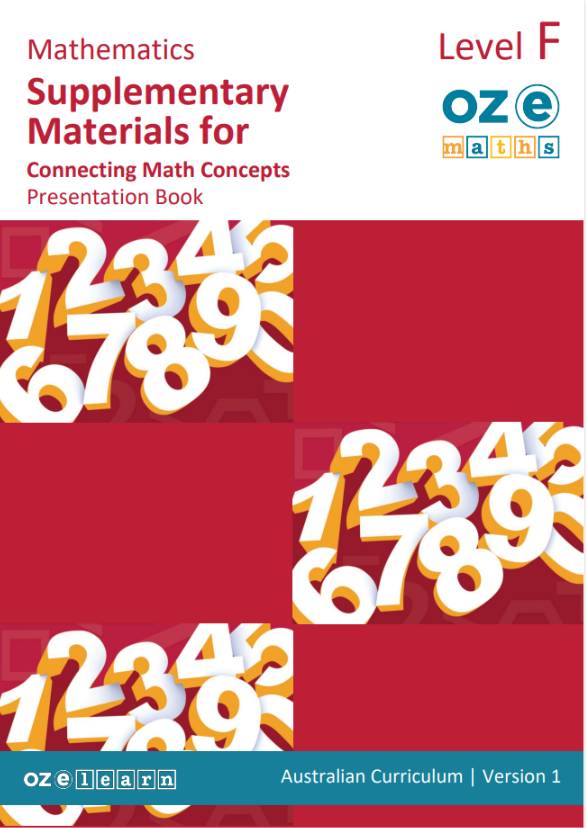## Oz-e-maths Supplementary Materials for Connecting Maths Concepts Years F-5

Top-ups and Swap-outs for Currency and Measurement - Year 5### Teaching resources

Overview
Top-ups and Swap-outs for Currency and Measurement – Year 5 is a Mathematics unit for Year 5 students. It works in cooperation with Connecting Maths Concepts (CMC) to ensure that delivery of CMC aligns with Australian Curriculum gap requirements. It aligns to the Australian Curriculum:
• Select and use appropriate displays or visualisations, interpret and communicate findings or solutions within the context (AC9M5ST03).
• Justify choices and explain results in terms of the situation (AC9M5N09).
• The outcome in this lesson is now a Year 6 outcome
• Use observation and experience to assign probabilities that events occur in a given context, using fractions, percentages and decimals to indicate their estimated likelihood (AC9M6P01).
• Recognise and describe what changes and what remains the same under the transformation and identify any rotational symmetries (AC9M5SP03).
• Create simple financial plans (ACMNA106).
Learning Objectives
In Lesson 20 (CMC Level F: Lesson 31-40), students learn to:
• use the grid reference system to describe location
• apply directional language using the compass points (North, East, South and West)
• use a legend to identify landmarks on a map
• construct a legend from the information contained in a map, including a compass.
In lesson 21 (CMC Level F: Lessons 41-50), students learn to:
• define data and how it can be displayed
• examine data question types
• identify and use open questions to closed questions
• identify categorical and numerical data types
• collect categorical data and conduct a survey.
In Lesson 23 (CMC Level F: Lesson 61-70), students learn to:
• calculate a tuckshop order
• define and calculate a budget
• calculate a budget
• create a budget for a fundraising event
• create a budget for a class fundraising event
• define and identify GST on items.
In Lesson 25 (CMC Level F: Lesson 81-90), students learn to:
• define probability - certain, likely, even chance, unlikely and impossible - and use these terms to describe the probability of an event occurring
• describe the probability of events occurring using certain, likely, even chance, unlikely and impossible
• recognise that probabilities can be expressed as a fraction from 0 to 1.
In Lesson 28 (CMC Level F: Lesson 111-120), students learn to:
• define and identify the rotational symmetry of two-dimensional shapes
• identify the points of rotational symmetry
• identify rotational symmetry in patterns
• identify reflection symmetry using the x or y axis to complete the shape
• identify line symmetry by drawing a reflection
• define translation and use this term to describe two-dimensional shapes
• use translation to describe two-dimensional shapes
• describe the translations, reflections, or rotations of two-dimensional shapes
• use rotational symmetry to draw a two-dimensional shape
• define transformation and identify translations, reflections, and rotations
• use enlargement transformation to draw two-dimensional shapes.
Success Criteria
Top-Ups
• Apply directional language and understand the compass points.
• Collect and identify categorical and numerical data types.
• Understand budgeting.
• Define probability using language and fractions.
• Understand symmetry, transformations, translations, reflections, or rotations of two-dimensional shapes.
Swap-Outs
• Apply arithmetic skills for all units of measurement.
• Solve comparison word problems.
Assessment
Mastery Tests
• Mastery Tests are given at the end of every 10 lessons, designed to be taught over a two-week cycle. Mastery Tests allow teachers to monitor student understanding of the concepts taught in previous lessons and identify where reteaching is needed.
• The Teaching Guide contains the Mastery Test script. Mastery Tests are delivered using a presentation script, board displays and a handout for students. The Teaching Guide also contains the Answer Key.

Cumulative Tests

• There are two Cumulative Tests for Swap-Outs that are completed after lesson 60 and lesson 120. The Cumulative Tests are in the Teaching Guide.
• Teachers copy the assessment and distribute to students at testing time.

## Recommended Units

\Learnworlds\Codeneurons\Pages\ZoneRenderers\CourseCards